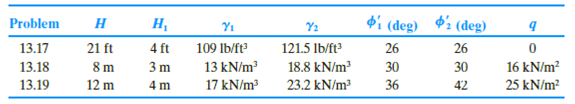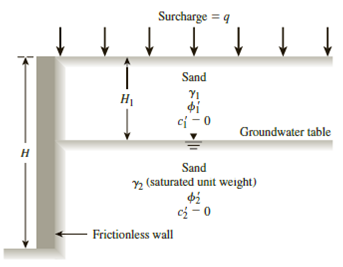Chapter 13, Problem 13.18PPrinciples of Geotechnical Enginee...

9th Edition
Braja M. Das + 1 other
ISBN: 9781305970939

Solutions

Chapter
SectionPrinciples of Geotechnical Enginee...

9th Edition
Braja M. Das + 1 other
ISBN: 9781305970939
Textbook Problem

13.17 through 13.19 A retaining wall is shown in Figure 13.41. For each problem, determine the Rankine active force, Pa, per unit length of the wall and the location of the resultant.Figure 13.41

To determine

Find the Rankine active force Pa per unit length of the wall and the location z¯ of the resultant force.

Explanation

Given information:

The height (H) of the retaining wall is 8.0 m.

The depth H1 of sand is 3.0 m.

The unit weight γ1 of the sand is 13kN/m3.

The sand friction angle ϕ1 is 30°.

The saturated sand friction angle ϕ2 is 30°.

The cohesion c1  of sand is 0.

The surcharge pressure (q) is 16kN/m2.

The depth H2 of saturated sand is 4.0 ft.

The saturated unit weight γ2 of the sand is 18.8kN/m3.

The cohesion c2 of saturated sand is 0.

Calculation:

Determine the active earth pressure coefficient Ka using the formula.

Ka=tan2(45ϕ2)

Substitute 30° for ϕ.

Ka=tan2(4530°2)=tan2(4515)=tan2(30)=0.333

Determine the total stress σo at 0 m depth using the relation.

σo=q

Substitute 16kN/m2 for q.

σo=16kN/m2

Determine the pore water pressure at 0 m depth using the relation.

u=γw×h

Here, γw is the unit weight of the water.

Take the unit weight of the water as 9.81kN/m3.

Substitute 9.81kN/m3 for γw and 0 m for h.

u=9.81×0=0

Determine the effective active earth pressure σa at 0 m depth using the relation.

σa=σoKa

Substitute 16kN/m2 for σo and 0.39 for Ka.

σa=16(0.333)=5.32kN/m2

Determine the total stress σo at 3 m depth using the relation.

σo=q+γ1×H1

Substitute 16kN/m2 for q, 13kN/m3 γ1, and 3.0 m for H1.

σo=16+13×3.0=55kN/m2

Determine the pore water pressure at 3.0 m depth using the relation.

u=γw×h

Substitute 9.81kN/m3 for γw and 0 m for h.

u=9.81×0=0

Determine the effective active earth pressure σa at 3.0 m depth using the relation.

σa=σoKa

Substitute 55kN/m2 for σo and 0.333 for Ka.

σa=55(0.333)=18.31kN/m2

Determine the total stress σo at 8 m depth using the relation.

σo=q+γ1×H1+(γ2γw)×H2

Substitute 16kN/m2 for q, 13kN/m3 γ1, 3.0 m for H1, 18.8kN/m3 γ2, 9.81kN/m3 for γw, and 5.0 m for H2.

σo=16+13×3+(18.89.81)×5=99.95kN/m2

Determine the pore water pressure at 8 m depth using the relation.

u=γw×h

Substitute 9.81kN/m3 for γw and 5.0 m for h.

u=9.81×5=49.05kN/m2

Determine the effective active earth pressure σa at 8 m depth using the relation

Still sussing out bartleby?

Check out a sample textbook solution.

See a sample solution

The Solution to Your Study Problems

Bartleby provides explanations to thousands of textbook problems written by our experts, many with advanced degrees!

Get Started

What is a DBMS, and what are its functions?

Database Systems: Design, Implementation, & Management

Briefly define quality control and explain its purpose.

Precision Machining Technology (MindTap Course List)

Describe modular design, and explain the two main prototyping methods.

Systems Analysis and Design (Shelly Cashman Series) (MindTap Course List)

What are the steps in the SDLC?

Enhanced Discovering Computers 2017 (Shelly Cashman Series) (MindTap Course List)

What metals can be cut with the oxyfuel gas process?

Welding: Principles and Applications (MindTap Course List)